# SMPM_MTPA

Test example: PermanentMagnetSynchronousMachine, investigating maximum torque per Amps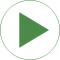# Information

This information is part of the Modelica Standard Library maintained by the Modelica Association.

This example investigates the maximum torque per amps (MTPA) of a quasi static permanent magnet synchronous machine. The machines is operated at constant speed. The current magnitude is kept constant and the current angle is rotated from 0 to 360 degrees with the simulation period of one second.

In this simulation the angle is the following angles are calculated:

• `phi_v` = angle of voltage phasor
• `phi_i` = angle of current phasor
• `phiphi_v - phi_i` = angle between voltage and current phasor
• `theta` = rotor displacement angle
• `epsilon = phi - theta` = current angle

Simulate for 1 second and plot (versus angle epsilon):

• `smpmQS.tauElectrical`: machine torque
• `smpmQS.abs_vs`: machine phase voltage magnitude
• `phi`: phase angle between voltage and current phasor
##### Note

The resistors connected to the terminals of the windings of the quasi static machine model are necessary to numerically stabilize the simulation.

# Parameters (10)

VNominal Value: 100 Type: Voltage (V) Description: Nominal RMS voltage per phase Value: 50 Type: Frequency (Hz) Description: Nominal frequency Value: 50 Type: Frequency (Hz) Description: Actual frequency Value: 1 Type: Time (s) Description: Frequency ramp Value: 181.4 Type: Torque (N·m) Description: Nominal load torque Value: 1.2 Type: Time (s) Description: Time of load torque step Value: 0.29 Type: Inertia (kg·m²) Description: Load's moment of inertia Value: 2 * pi * fNominal / smpmData.p Type: AngularVelocity (rad/s) Description: Nominal angular velocity Value: false Type: Boolean Description: Use positive range of angles, if true Value: Description: Machine data

# Outputs (5)

theta Default Value: rotorAngleQS.rotorDisplacementAngle Type: Angle (rad) Description: Rotor displacement angle, quasi static Default Value: Modelica.Math.wrapAngle(smpmQS.arg_is, positiveRange) Type: Angle (rad) Description: Angle of current Default Value: Modelica.Math.wrapAngle(smpmQS.arg_vs, positiveRange) Type: Angle (rad) Description: Angle of voltage Default Value: Modelica.Math.wrapAngle(phi_v - phi_i, positiveRange) Type: Angle (rad) Description: Angle between voltage and current Default Value: Modelica.Math.wrapAngle(phi - theta, positiveRange) Type: Angle (rad) Description: Current angle

# Components (18)

smpmData smpmQS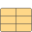Description: Machine data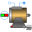Type: SM_PermanentMagnet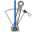Type: ConstantSpeed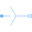Type: Star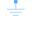Type: Ground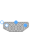Type: MultiTerminalBox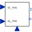Type: CurrentController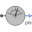Type: AngleSensor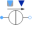Type: StarType: Ground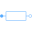Type: Resistor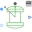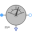Type: Star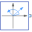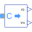Type: ComplexToReal